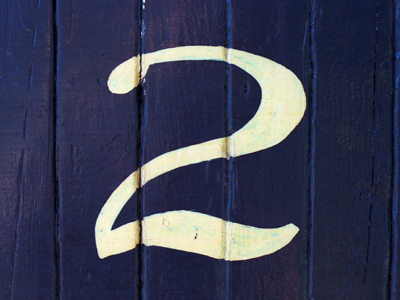If you can count in twos it will help you to multiply and divide by two as well.

# Year 2 Calculation - Multiplication and Division By 2

This quiz addresses the requirements of the National Curriculum KS1 Maths and Numeracy for children aged 6 and 7 in year 2. Specifically this quiz is aimed at the section dealing with multiplication and division by 2.

Counting in 2s is the earliest multiplication skill taught which also helps children when dividing. It is usually practised regularly, in order for the skill to be fluent, and children are also taught to recognise that multiples of two are even. Once the 2 times table is familiar, children will learn how to use the multiplication facts to solve division questions. For example, if they know that 2 x 6 = 12, then they may also recognise that 12 ÷ 6 = 2 or 12 ÷ 2 = 6

Question 1
I know that 2 x 15 = 30. What else do I know?
30 x 2 = 15
2 x 15 = 20
30 x 15 = 2
30 ÷ 15 = 2
If 2 groups of 15 makes 30, then 30 divided into 15 groups will mean 2 in each group
Question 2
Doubling is the same as...
dividing by 2
halving
subtracting over and over
To double 10, you would add 10 to itself: 10 + 10 = 20
Question 3
What is the next number in this sequence: 2, 4, 6, 8, 10, ?
14
11
12
20
The numbers are going up in twos
Question 4
Is 45 in the 2 times table?
No, because it is an odd number
Yes, because it is an even number
Yes, because it has a 4 at the beginning
No, because it is too big
Multiples of 2 end with 2, 4, 6, 8 or 0
Question 5
What is half of 60?
2
20
3
30
Finding half is the same as dividing by 2. Knowing 6 ÷ 2 = 3 helps with 60 ÷ 2 = 30
Question 6
How many 2s are there in 28?
16
14
10
8
Counting up in 2s until you reach 28 is helpful, as is halving 20 and halving 8
Question 7
Which is the odd fact out?
6 x 2 = 12
12 ÷ 6 = 2
12 x 2 = 6
12 ÷ 2 = 6
12 x 2 = 24 not 6!
Question 8
10
25
50
12
5 x 2 = 10
Question 9
I put 20 children into pairs. How many pairs can I make?
2, because 20 ÷ 10 = 2
5, because 20 ÷ 10 = 5
20, because 20 ÷ 2 = 20
10, because 20 ÷ 2 = 10
We also know that 10 x 2 = 20
Question 10
What is 20 x 2?
22
40
10
12
Multiplying by 2 is the same as doubling a number